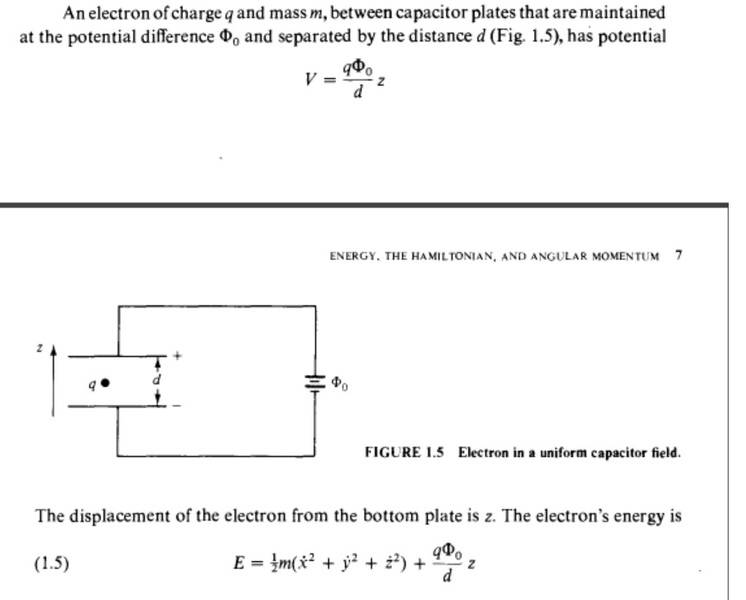# Electric potential of an electron in a capacitor

• user35711
Yes, potential energy is negative when you start with the top plate and then goes to zero as you reach the bottom plate.

#### user35711

Why is the electric potential of an electron in a capacitor measured from the negative plate and not the positive plate here?
This is from Liboff Introductory quantum mechanics 1st(current is 4th) edition:I don't understand why the distance z is measured from the bottom plate if the potential is of an electron which should gain Energy going to the top plate.

#### Attachments

In the equations the q is welcome to be positive or negative. When you plug in the negative charge of the electron I think you will see the equations make sense with the indicated direction of z.

There is no particular reason they had to choose z as up, and had they chosen the other direction the equations could be corrected with a minus sign. However up is a perfectly fine choice and the equations are written correctly for that choice.

•sophiecentaur
Cutter Ketch said:
However up is a perfectly fine choice
Direction choice is quite arbitrary as long as you stick with it. Students are often confused by a similar thing in basic problems of acceleration under gravity - when you drop an object, do you choose distance along its downward path to be positive (the way it's actually going) or negative (because up is often positive)? The answer will be correct, whichever you choose.

It's not the positive or negative sign that doesn't make sense. The complimentary value of the potential is given. If you measure from the bottom, you are giving a different value for the potential energy than when you measure from the top which is the distance potential the electron can accelerate through.

Agreed, there will be two values of potential energy, depending which plate you choose to be zero (reference). But the work done shifting the charge from one point to another will be equal to the Potential Difference, which will give you the same answer, whichever your axes are.
Potential is not defined in terms of "accelerating" an electron - it's the work done in moving the charge from a chosen point. I'm not quite sure where you problem lies but is it something to do with the distance traveled from start position to one plate or the other? You will get two different magnitudes and two different signs for this if not starting from the mid point.

You're right, I meant to say the energy in joules after moving charge q, not acceleration.

user35711 said:
You're right, I meant to say the energy in joules after moving charge q, not acceleration.
OK - so wouldn't you expect two possible results, depending on which side the charge ends up? Is it not just that simple?

What I didn't understand but now think I do is that the potential energy is negative when you start with the top plate and then goes to zero as you reach the bottom plate with the coordinates chosen.

## 1. What is the electric potential of an electron in a capacitor?

The electric potential of an electron in a capacitor is the amount of electrical energy that an electron possesses due to its position in the capacitor. It is measured in volts (V).

## 2. How is the electric potential of an electron in a capacitor calculated?

The electric potential of an electron in a capacitor can be calculated using the formula V = Q/C, where V is the electric potential, Q is the charge on the capacitor, and C is the capacitance of the capacitor.

## 3. How does the electric potential of an electron in a capacitor change with distance?

The electric potential of an electron in a capacitor is inversely proportional to the distance between the plates of the capacitor. This means that as the distance increases, the electric potential decreases, and vice versa.

## 4. What is the relationship between the electric potential of an electron in a capacitor and the electric field?

The electric potential of an electron in a capacitor is directly proportional to the strength of the electric field between the plates of the capacitor. This means that as the electric field increases, the electric potential also increases.

## 5. How does the electric potential of an electron in a capacitor affect the movement of electrons?

The electric potential of an electron in a capacitor determines the direction and speed of electron movement. Electrons will move from a higher electric potential to a lower electric potential, and the greater the difference in potential, the faster the electrons will move.